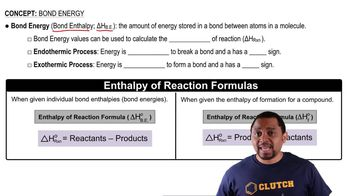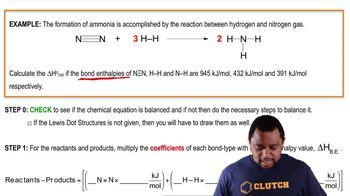Start typing, then use the up and down arrows to select an option from the list.
7:25 minutes
Problem 118a
Textbook Question

# Calculate an approximate heat of combustion for ethane (C2H6) in kilojoules by using the bond dissocation energies in Table 9.3. (The strength of the O“O bond is 498 kJ/ mol, and that of a C “ O bond in CO2 is 804 kJ/mol.)Verified Solution
This video solution was recommended by our tutors as helpful for the problem above.
244views